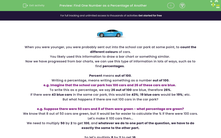# Find One Number as a Percentage of Another

In this worksheet, students will find percentages of one number out of a total with and without a calculator, which may or may not require conversion so it can be equated to 100.Key stage:  KS 4

Year:  GCSE

GCSE Subjects:   Maths

GCSE Boards:   AQA, Eduqas, OCR, Pearson Edexcel,

Curriculum topic:   Ratio, Proportion and Rates of Change, Fractions, Decimals and Percentages

Curriculum subtopic:   Ratio, Proportion and Rates of Change Percentages

Difficulty level:#### Worksheet OverviewWhen you were younger, you were probably sent out into the school car park at some point, to count the different colours of cars.

You likely used this information to draw a bar chart or something similar.

Now we have progressed from bar charts, we can use this type of information in lots of ways, such as to find percentages

Percent means out of 100.

Writing a percentage, means writing something as a number out of 100.

e.g. Imagine that the school car park has 100 cars and 26 of these cars are blue.

To write this as a percentage, we say 26 out of 100 are blue, therefore 26%.

If there were 43 blue cars in the same car park, this would be 43%; 19 blue cars would be 19%; etc.

But what happens if there are not 100 cars in the car park?

e.g. Suppose there were 50 cars and 8 of them were green - what percentage are green?

We know that 8 out of 50 cars are green, but it would be far easier to calculate the % if there were 100 cars.

Let's make it 100 cars then...

We need to multiply 50 by 2 to get 100, and whatever we do to one part of the question, we have to do exactly the same to the other part.

So let's multiply 8 by 2 to get 16.

This is easier now...

16 out of 100 cars are green, so that is 16%.

If we cancel down 16 out of 100, we get back to 8 out of 50, so we know this is correct.Take note: You many also need to cancel the 'out of ' down to reach 100.

e.g. What is 52 out of 400 as a %?

We would divide both numbers by 4 (as 400 ÷ 4 = 100) to get 13 out of 100  = 13%.

Now for the tricky part...

The 'out of' may not be that simple to convert.

e.g. What would we do if we wanted to write 8 out of 32 as a percentage?

32 obviously does not multiply up to 100.

Could we multiply it up to 200?

The best way to find out is divide 200 by 32 to see if it goes into it equally.

What did you get?

A decimal number, not helpful really.

Let's try another option... how about 300

Another decimal, not great.

Keep going until it works... try 400, 500, etc.

In this case, our best bet is 800 - phew! I thought we were never going to get there!

800 divided by 32 = 25

Now multiply 8 by 25 which gives us 200.

We now have 200 out of 800.

To turn 800 into 100, we need to divide by 8 and then do the same to the 200.

Did you get 25%?

Magic!In this activity, we will find percentages of one number out of a total which we may or may not need to convert so we can equate it to 100.

Look out for a magical tip halfway through to give you an alternative method and a speedy shortcut!

You may want to have a calculator handy so that you can concentrate on practising these methods and not stretching your mental maths brain.

### What is EdPlace?

We're your National Curriculum aligned online education content provider helping each child succeed in English, maths and science from year 1 to GCSE. With an EdPlace account you’ll be able to track and measure progress, helping each child achieve their best. We build confidence and attainment by personalising each child’s learning at a level that suits them.

Get started